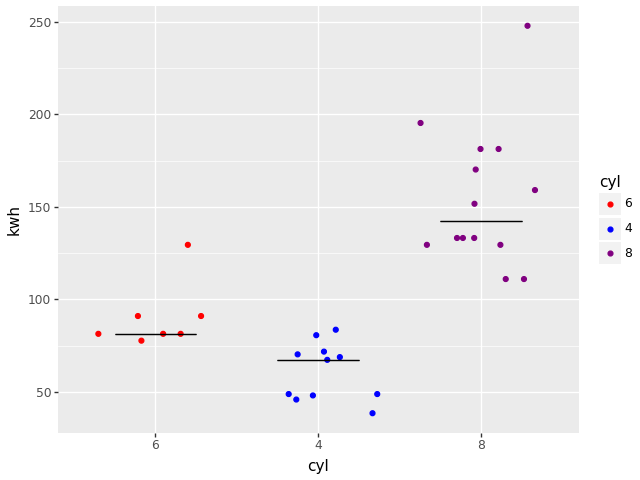Combines plotnine and dppd

# dppd_plotnine

dppd_plotnine combines the power of plotnine and dppd

It allows you to use code like this

   import numpy as np
from dppd import dppd
import dppd_plotnine
from plotnine.data import mtcars
import plotnine as p9
dp, X = dppd()

plot = (
dp(mtcars)
.assign(kwh=X.hp * 0.74)
.categorize("cyl")
.p9()
"cyl",
"kwh",
color="cyl",
position=p9.position_jitter(height=0, random_state=500),
)
x="cyl",
y="kwh_median",
ymin="kwh_median",
ymax="kwh_median",
data=dp(X.data)
.groupby("cyl")
.summarize(("kwh", np.median, "kwh_median"))
.pd,
)
.scale_color_manual(
["red", "blue", "purple"]
)  # after pd, X is what it was before
.pd
)
plot.save("test.png")Please see our full documentation at https://dppd_plotnine.readthedocs.io/en/latest/ for more details of the straight and enhanced plotnine mappings available.

## Project details

This version0.2.50.2.40.2.30.2.20.2.10.20.1

Uploaded source